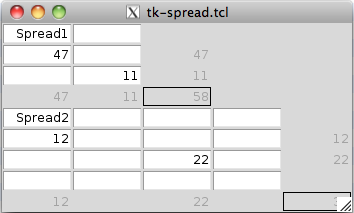View · Search · Index
No registered users in community xowiki
in last 10 minutes

A small Spreadsheet implementation, originally developed by Richard Suchenwirth in plain Tcl (see http://wiki.tcl.tk/1287). The spreadsheet was rewritten in an object oriented manner as a design study in NX by Gustaf Neumann in May 2011.```package require Tk
package require nx::trait

##############################################################################
#
# The SpreadSheet computes simply totals for rows and columns.
##############################################################################
#
# The following attributes can be used for configuring the
#
:property {rows:integer 3}
:property {cols:integer 2}
:property {width:integer 8}

#
# If no widget is provided, use the name of the object as widget
# name.
#
:property {widget ".[namespace tail [self]]"}

#
# Use the nx callback trait
#
:require trait nx::trait::callback

#
# The method "cell" hides the internal respresentation and sets a
# cell to a value.
#
:method cell {pair value} {
set :data(\$pair) \$value
}

#
# The constructor builds the SpreadSheet matrix via multiple text
# entry fields.
#
:method init {} {
set :last \${:rows},\${:cols}  ;# keep grand total field
trace var [:bindvar data] w [:callback redo]
frame \${:widget}
for {set y 0} {\$y <= \${:rows}} {incr y} {
set row [list]
for {set x 0} {\$x <= \${:cols}} {incr x} {
set e [entry \${:widget}.\$y,\$x -width \${:width} \
-textvar [:bindvar data(\$y,\$x)] -just right]
if {\$x==\${:cols} || \$y==\${:rows}} {
\$e config -state disabled -background grey -relief flat
}
lappend row \$e
}
grid {*}\$row -sticky news
}
\$e config -relief solid
}

#
# The method "redo" is triggered via the updates in the cells
#
:public method redo {varname el op} {
if {\$el ne \${:last}} {
lassign [split \$el ,] y x
if {\$x ne ""} {
:sum \$y,* \$y,\${:cols}
:sum *,\$x \${:rows},\$x
} ;# otherwise 'el' was not a cell index
}   ;# prevent endless recalculation of grand total
}

#
# The method "sum" adds the values matched by pattern (typically a
# row or column) and sets finally the target column with the total
#
:method sum {pat target} {
set sum 0
set total "" ;# default if no addition succeeds
foreach {i value} [array get :data \$pat] {
if {\$i != \$target} {
if {[string is double -strict \$value]} {
set total [set sum [expr {\$sum + \$value}]]
}
}
}
:cell \$target \$total
}
}```

```SpreadSheet create x {
# populate with some values
:cell 1,0 47
:cell 2,1 11
}```

```SpreadSheet create y -rows 4 -cols 4 {
`pack [x cget -widget] [y cget -widget] -fill both`